#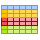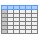Group & Ungroup

The Group function is used to create virtual groups (sub-tables) according to selected categorical variables. Consequently, you can perform other applied functions within these groups, such as functions from Subset or Summarize set. Conversely, the Ungroup function allows you to cancel the previous grouping and continue to work with the entire dataset.

## Properties

 Group By Variables This property allows you to define variables from the dataset by which the dataset records are grouped.

The Ungroup function has no additional properties.

## Keywords

Group, Ungroup, Table Grouping, Excel Group By, Group By Excel Alternative, Computation in Groups, Summation in Groups, Summarize in Groups, Remove Grouping

## How to Process and Summarize Excel Data in Groups

Often, we need to process larger amounts of data (thousands of rows) in Excel, such as the sum of sales grouped by countries and months of the year. An example of this dataset is shown in the following figure.In SQL, this can be done smoothly and quickly with the Group By command. Reshape.XL brings this functionality in a very simple and effective form. Grouping implementation in Reshape.XL is a bit different and brings several benefits. Within a set of data processing functions, you can dynamically change the grouping settings or completely disable it for the part of processing.

For a better understanding, I will give an example. Imagine a product sales database that includes product categories, order pricing and date of processing. We want to add a column with the average order price for each year. For analytical purposes, we want to choose the Top 20 orders in each product category in each year. Consequently, we want to select all the orders that exceed the average value in each year. This type of processing is relatively complicated and in the case of SQL, we have to create several SQL selections and connect them together. In the Reshape.XL add-in this is done within one process without the need to link intermediate results.

For the grouping application, we use the first two buttons in the ribbon toolbar tab Reshape - Group and Ungroup.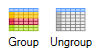Their functionality is shown in the following example. We want to calculate average values for Q, T and Tvz variables. This summarization is proceed using the Sum_Mean function. In the properties panel, we select the variables we want to use. After click on the Run button we see in the table average values of the selected variables for the entire dataset.If you want these average values calculate for groups according to the selected categorical variable, insert the Group function before the Sum_Mean. In the Properties Panel for this function, we select the variable that represents individual categories for which we will count the averages. In the following example, we group the dataset by variable region. Then, after pressing the Run button, the average values will be calculated for each region.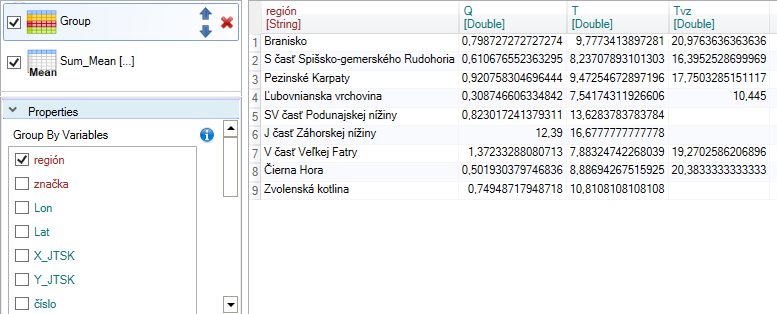Another example will be a bit more complicated. In this case, we have an input table with two variables. One variable contains categorical values and the second are numerical values.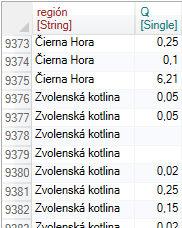Our task is to add two new columns that will include the average value for the entire dataset and the average values for each category. The procedure is shown in the following figure. In the first step, we selected the two described variables from the larger dataset using the Select function.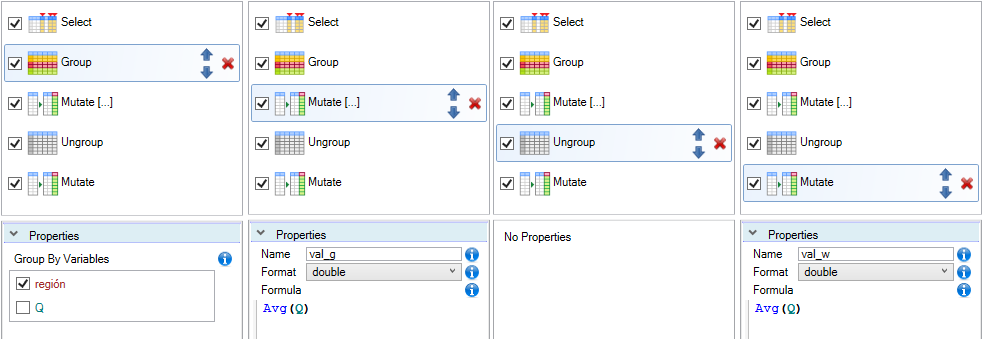In the second step, we grouped the dataset using the (categorical) variable - region. After this step, all calculations are executed in individual "virtual" sub-tables. If the function is performed individually for each group, this is marked by the "[...]" postfix.

Subsequently, we created a new column using the Mutate function by formula - Avg(Q) which calculates the average value for each category. The result was saved in a new column named val_q. Then we want to get average value of the Q variable for the entire dataset (without grouping). For this purpose, we have inserted the Ungroup function into the sequence of commands, which deactivates the previous grouping.

After that, we added the Mutate function again with the same formula. Since grouping was deactivated, the calculation is proceed across the entire dataset and the result was inserted into the new variable val_w.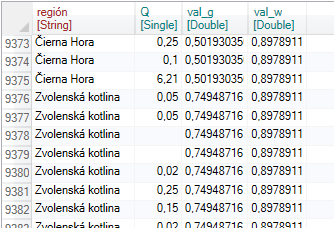If we use another tool, we would get this output in much more complicated approach. The great advantage of Group and Ungroup functions in Reshape.XL is that you can combine these functions in one sequence optionally and perform relatively advanced calculations in a simple and effective way.

The Group and Ungroup functions have in Reshape.XL great importance, because fundamentally changes the nature of the function execution process. If the selected function is affected by grouping, this is displayed using the postfix "[...]" after the function name. The impact of grouping is individually described in the documentation for each function.